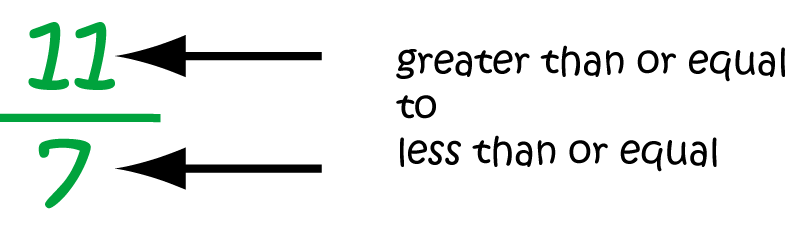# Definition of Improper FractionAn improper fraction is a fraction in which the numerator (top number) is bigger than or equal to the denominator (bottom number).

Improper fractions can be converted to mixed numerals. For example, $\dfrac{15}{8}$ is equal to the mixed numeral, $1 \; \dfrac{7}{8}$.

There is nothing wrong with improper fractions. They're often necessary when you're calculating with fractions, but sometimes you can get a better idea of how big something is by converting it from an improper fraction into a mixed numeral.

### Description

The aim of this dictionary is to provide definitions to common mathematical terms. Students learn a new math skill every week at school, sometimes just before they start a new skill, if they want to look at what a specific term means, this is where this dictionary will become handy and a go-to guide for a student.

### Audience

Year 1 to Year 12 students

### Learning Objectives

Learn common math terms starting with letter I

Author: Subject Coach
You must be logged in as Student to ask a Question.